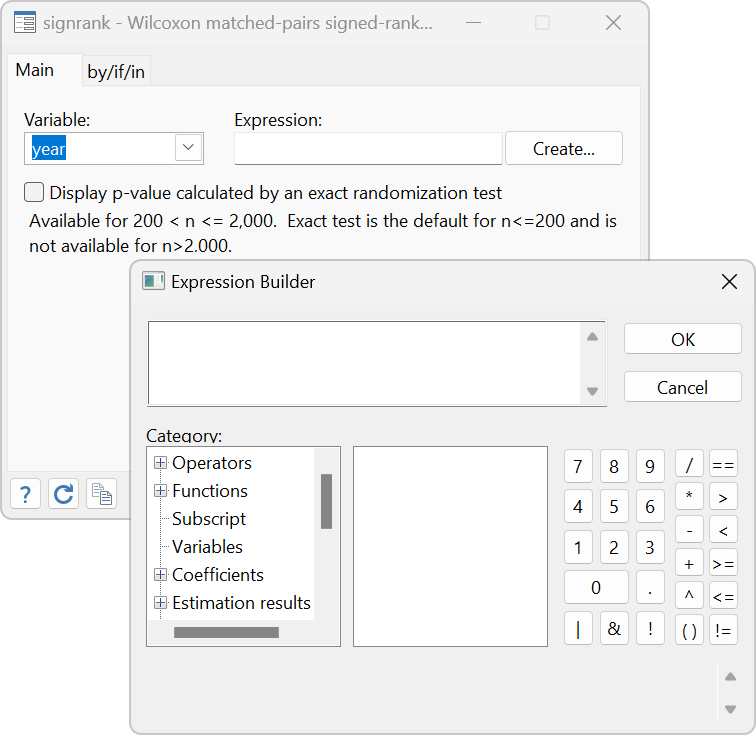Nonparametric tests

Sign test

Wilcoxon signed-rank testWilcoxon rank-sum (Mann–Whitney) test

Equality of medians

Kruskal–Wallis test

Kolmogorov–Smirnov test

Binomial probability test

Chi-squared tests for association

Tests for trend

One- and two-sample tests of proportions

Test for random order

Equality of survivor functions

Equality of ROC areas

Equality of ROC area against a standard ROC curve

CUSUM

Interrater agreement

Quantile regression

Median regression

Least absolute deviations regression

User-specified quantiles

Koenker and Bassett and Huber sandwich standard errors with many choices of bandwidth methods and kernels

Simultaneous quantile regression

Interquartile range regression

Nonparametric correlation coefficients

Spearman’s rank order

Kendall’s rank order

Nonparametric regression

Multiple covariates supported

Continuous covariates (8 kernels available)

Discrete covariates (2 kernels available)

Techniques

local constant regression

local linear regression

Point estimates with SEs and CIs

Derivative estimates with SEs and CIs

Automatic optimal bandwidth selection

Interface to margins for advanced inference

Estimates of population-averaged and subpopulation-averaged means and effects

Fully conditional means and effects at any specified values of the covariates

Confidence intervals

Tests

Graphs via marginsplot

Watch Nonparametric regression.

Survival analysis

Kaplan–Meier curves

Nelson–Aalen curves

Logrank and other tests of equality

Treatment effects

Nearest-neighbor matching

Propensity-score matching

Resampling and simulations

Bootstrap sampling and estimation

Sampling with replacement

Monte Carlo simulations

Smoothing

Univariate kernel density estimation

Lowess smoothing

Kernel-weighted local polynomial smoothing

Robust nonlinear smoother

ROC analysis